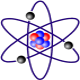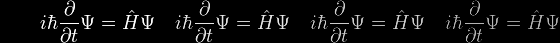Well, what's been happening in the world of molecular integrals since my researches wound down in 1976? Quite a lot, it would appear...

A great many papers about the Barnett-Coulson / Lowdin expansions and their application to multi-centre integrals over Slater-type atomic orbitals have in fact appeared since that time. Whether everybody has been reinventing the wheel I cannot say; perhaps genuinely new and improved formulations have been found, but my instinct (as stated earlier) is that efficient structuring of the computer implementation, plus effective use of symmetry, plus parallel processing, are probably of equal or greater importance.

Being no longer affiliated to an academic organisation, I can only establish the existence of relevant research papers by means of (say) Google in the first instance. Their titles and abstracts are accessible this way, but not their detailed content. For that I must either pay quite a substantial online viewing fee, or else visit the local University library, which doesn't really carry any hard-copy journals in this specialist area. So to be honest, I'm not really much the wiser at the moment - but I'd be very interested to receive feedback from any of you out there who are actively researching this area.

It should be mentioned at this point that the Barnett-Coulson expansion coefficients were traditionally called zeta functions, whereas in the Lowdin formalism they are referred to as alpha functions. Clearly the two must be essentially equivalent, but the latter terminology now seems to be the norm.

The names of A Bouferguene, H W Jones, R R Sharma and N Suzuki seem to be particularly prominent. I have itemised some of their publications below, and in the case of Suzuki whose abstracts are particularly detailed I have included these as well, to give a flavour of his investigations.

(Disclaimer: In some of the following mathematical expressions, the builder of this web page has been uncertain which symbol correctly renders certain characters and has tentatively replaced them with a hash symbol (#). Readers are urged to study the original abstracts directly from the publishers. If you can ascertain what the characters should be, please send an email and this page will be updated accordingly.)

Bouferguene A, Jones H W,
J. Chem. Phys. 109, pp 5718-5729, 1998
"Convergence analysis of the addition theorem of Slater orbitals and its application to three-center nuclear attraction integrals"

Bouferguene A,
J Physics A, Vol. 38, No. 13, pp 2899-2916, 2005
"Addition theorem of Slater type orbitals: a numerical evaluation of Barnett-Coulson / Lowdin functions"

Bouferguene A, Safouhi H,
J Physics A, Vol. 39, No. 3, pp 499-511, 2006
"A complexity analysis of the Gauss-Bessel quadrature as applied to the evaluation of multi-centre integrals over STFs"

Bouferguene A, Safouhi H,
Int J Quant Chem, Vol. 106, Issue 11, pp 2398-2407, 2006
"Gauss-Bessel Quadrature: A tool for the evaluation of Barnett-Coulson / Lowdin functions"

Duret S, Bouferguene A, Safouhi H,
J Comput Chem, 29(6), pp 934-944, 2008
"Strategies for an efficient implementation of the Gauss-Bessel quadrature for evaluation of multicenter integrals over STFs"

Jones H,
Int J Quant Chem, Vol. 41, pp 749-754, 1992
"Lowdin Alpha Function, Overlap Integral, and Computer Algebra"

Jones H, Weatherford C,
J Mol Struct, Vol. 199, pp 233-243, 1989
"The Lowdin alpha function and its application to the multi-center molecular integral problem over Slater-type orbitals"

Macdonald, J.R., Dolding, R.M.
Molecular Physics, Vol. 31, Number 1, pp 289-293, 1976
"Analytic expressions for multi-centre integrals using Slater-type orbitals"

J. Math. Phys. 25, 1133 (1984); doi:10.1063/1.526256 (6 pages)

### Simple calculation of Löwdin's alpha-function

Noboru Suzuki
Department of Materials Science, The University of Electro-Communications, Chofu-Shi, Tokyo 182, Japan
(Received 27 July 1983; accepted 30 September 1983)

A function of the type f(R)YML(Θ,Φ) around a specific center B is expanded about another center A as f(R)YML(Θ,Φ) =∑#= ‖ M(1/r#(fLM ‖ a,r) x YM#(ϑ,φ), where YML(Θ,Φ) and YM#(ϑ,φ) are the complex spherical harmonics around the respective centers, which have been assumed to be quantized in the direction of A to B. The distances from the respective centers are R and r, and the separation between the two centers is a. The expansion coefficient α#( fLM ‖ a,r), which is usually called Löwdin's α-function, can be expressed in a remarkably simple form by using Silverstone and Moats' formula. Owing to the simplicity, the properties of the coefficients bK k(LM ‖ #) appearing in the α-function are manifested, and the recurrence formulas for evaluating bK k(LM ‖ #) are derived. Furthermore, when r≊0, the asymptotic expression for the α-function is given in a compact form.

J. Math. Phys. 26, 3193 (1985); doi:10.1063/1.526648 (7 pages)

### Simple calculation of Löwdin's alpha function. II. Easier procedure for evaluating bK k(LM ‖ #), and vanishing of hn,2n-i(LM ‖ #) for special values of i and n

Noboru Suzuki
Department of Materials Science, The University of Electro-Communications, Chofu-Shi, Tokyo 182, Japan
(Received 3 April, 1985; accepted 25 June 1985)

This paper subsequent to the one [J. Math. Phys. 25, 1133 (1984)] (referred to as Part I) presents the following new results: It is found out that for M=L and L-1 the coefficients bK k(LM ‖ #) in Löwdin's α-function have properties other than manifested in Part I. The expression for bK k(LM ‖ #) is shown to be equivalent to the one into which Sharma's expression, obtained in a different manner from that in Part I, is simplified by Rashid. The use of Rashid's expression leads to the recurrence formula for bK k(LM ‖ #) with respect to M only. This formula and the expression for the bK k(LM ‖ #) with M=L provide an easier procedure for successively evaluating bK k(LM ‖ #) than in Part I. Furthermore, it is proved that the coefficients hn,2n-i(LM ‖ #)> in the asymptotic form of the α-function vanish for i<#+M and for n<#.

J. Math. Phys. 31, 2314 (1990); doi:10.1063/1.528639 (13 pages)

### Simple calculation of Löwdin's alpha function. III. Procedure for calculating hn,2n-i(LM ‖ #)/(2n-i)! successively

Noboru Suzuki
Department of Applied Physics and Chemistry, The University of Electro-Communications, Chofu-Shi, Tokyo 182, Japan
(Received 30 October 1989; accepted 9 May 1990)

When r/a≊0, Löwdin's α function (1/r)α#(fLM ‖ a,r) is expressed as (1/r# ( fLMa,r) =2γ(LM ‖ #)aL-1n=# [∑imaxi=#+M[hn,2n-i (LM ‖ #)/(2n-i)!]a2n-ig(2n-i) (a)](r/a)2n-#, where a is a separation between a new center placed on the origin and the old center located at a given point on the z axis that is the origin of the coordinate system defining a function f(R)YML(Θ,Φ) to be expanded around the new center [f(R) is an arbitrary radial function, and YML(Θ,Φ), a complex spherical harmonics], and r is a distance from the new center. Here, imax=min{2n,2(L+#)}, and the function g(j)(a) is expressed as g(j)(a)=[(d/dR)(j) (f(iR)/RL-1)]R=a.

The closed form of the coefficients hn,2n-i(LM ‖ #) is given by Eq. (27) in J. Math. Phys. 26, 3193 (1985) (referred to as Part II). Since they are expressed in terms of the different coefficients bK k(LM ‖ #) independent of the form of f(R), hn,2n-i(LM ‖ #) constitute a set of universal constants as do bK k(LM ‖ #). The explicit expression for the factor γ(LM ‖ #) in front of the summation symbol is given by Eq. (2.9) in J. Math. Phys. 25, 1133 (1984). Hereafter the coefficients expressed by hn,2n-i(LM ‖ #)/(2n-i)! are symbolized by #n,2n-i(LM ‖ #). Although they are expressed in a complex form with a triple sum, that expression can be reduced to two considerably simple forms by changing the summation indices to others by using the addition theorem for binomial coefficients and the condition for the sum in this theorem to vanish. Moreover, in the two special cases that i=#+M and i=2(L+#) or 2(L+#)-1, those two expressions are reduced to two single-term forms, respectively.

Introducing into the expression for #n,2n-i(LM ‖ #) in terms of bK k(LM ‖ #) the recursion formula for bK k(LM ‖ #) in only M given by Eq. (24) in Part II, leads to the recursion formula for #n,2n-i(LM ‖ #) with respect to M. On the other side, the recursion formulas for #n,2n-i(LM ‖ #) with M=L and # as to i are obtained through a skillful manipulation. By connecting those recursion formulas for #n,2n-i(LM ‖ #) to each other, we can obtain a procedure for calculating #n,2n-i(LM ‖ #) successively. As the result of actual performance of the procedure, all the formulas expressing #n,2n-i(LM ‖ #) with restriction 0≤M≤min{L,#}≤max{L,#}≤2 are presented as functions of the parameter n in a table.

J. Math. Phys. 33, 4288 (1992); doi:10.1063/1.529831 (12 pages)

### Simple calculation of Löwdin's alpha function. IV. Procedure for evaluating the coefficients in expansion of a Slater-type atomic orbital

Noboru Suzuki
Department of Applied Physics and Chemistry, The University of Electro-Communications, Chofu-Shi, Tokyo 182, Japan
(Received 17 December 1991; accepted 10 July 1992)

By using the explicit expression for the coefficients bK k(LM ‖ #)[Eq. (16) in J. Math. Phys. 26, 3193 (1985), which is referred to hereafter as Part II], a closed expression for the coefficients cij(NLM ‖ #) appearing in Löwdin's alpha function (1/r#(NLMa,r) for a Slater-type atomic orbital is obtained. By manipulating its expression mathematically, it was manifested that cij(NLM ‖ #) vanishes if i≥N+L-M+#. For the specific cases that each of i and j takes 0 or 1, cij(NLM ‖ #) take the same value. From the symmetry relation on bK k(LM ‖ #) [Eq. (6) in Part II], it was proved that cij(NLM ‖ #) = cji(NLM ‖ #), provided M=L. Through some manipulation, the recursion formula for cij(NLM ‖ #) in N was derived, while that about M was obtained by introducing the recursion formula for bK k(LM ‖ #) in M [Eq. (24) in Part II] into the expression for cij(NLM ‖ #). For the special cases where N and M both are equal to L, the expression for cij(NLM ‖ #) is given in a compact and symmetric form. The use of this expression leads to a recursion formula for cij(LLL ‖ #) in L. Especially, cij(LLL ‖ #) for L=0 is expressed by a single term, and a recursion formula for cij(000 ‖ #) in # was obtained in a very simple form. The systematic use of these recursion formulas leads to a procedure for evaluating cij(NLM ‖ #) successively. Finally, cij(NLM ‖ #) evaluated by this procedure, with the restriction that 2≥NL≥0 and 2≥min{L,#}≥M≥0, are given in a matrix form.

Perhaps we can finish where we began, with the maestro himself - several of his recent publications, such as this one, seem to have revisited the topic of molecular integrals and his eponymous expansion:

Journal of Symbolic Computation
Volume 42, Issue 3, March 2007, Pages 265-289

### Mathscape and molecular integrals

Michael P. Barnett

Abstract

I have used MATHEMATICA to solve several problems that relate to the symbolic calculation of the 'molecular integrals' that are a mainstay of computational chemistry.

This work has provided many new results of chemical and mathematical interest, and it has led to a powerful programming methodology that I call MATHSCAPE that uses a novel open ended set of macros. Some further work on molecular integrals is presented here, largely as an introduction to MATHSCAPE. I discuss (1) the immediate mathematical problem of the 'J' integrals, (2) the key features of MATHSCAPE, (3) a novel reduction of the J integrals, (4) the chemical context of this work, and (5) the computer science context.